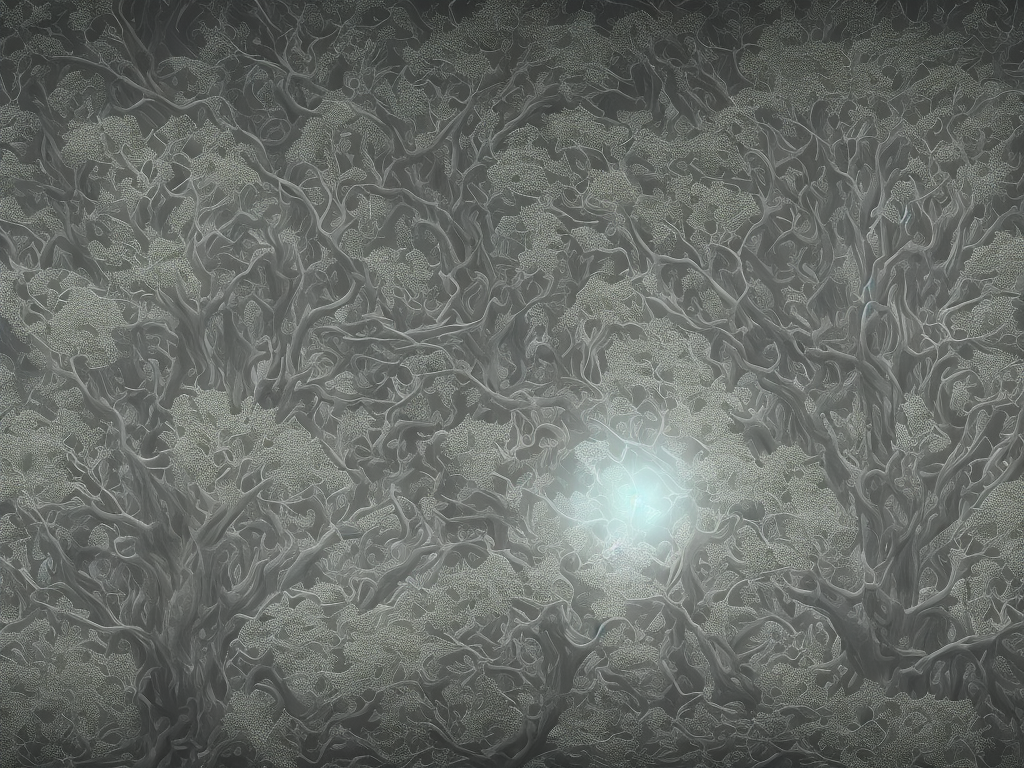# Difference Between Iteration And Recursion

tl;dr
Iteration is a repetitive process that involves doing the same task until a certain condition is met, while recursion involves breaking down a complex problem into smaller sub-problems and solving them one by one using the same function.As a computer programmer, it is essential to understand the two essential concepts that are commonly used to control program flow – iteration and recursion. Although these concepts may seem like ordinary programming structures, there are differences between them that are important to understand. The key difference between iteration and recursion lies in the way they solve a problem, and how they control program flow.

Iteration is a repetitive process that involves doing the same task over and over again until certain conditions are met. It is a process that involves a loop that performs a specific task as long as the condition is met. In other words, the task is executed repeatedly until a specific termination condition is met.

On the other hand, recursion involves breaking down a complex problem into smaller sub-problems and solving them one by one. These sub-problems are solved by calling the same function repeatedly until the base condition is met. Recursion is a technique in which a function calls itself repeatedly to solve a problem. Recursion is essentially solving a complex problem by breaking it down into simpler versions of itself.

Here are some differences between iteration and recursion that you need to know.

Function Calls

In recursion, the function calls itself until the base condition is achieved. With every function call, a new instance of the function is created, which is stored in the stack memory. Each instance of the function waits for its sub-problems to be solved before returning to the main function. Once the base condition is met, the functions start to return their values, and the result is obtained by adding up all the returned values.

Iteration, on the other hand, uses the loop to iterate over a series of instructions until the termination condition is met. Every instruction is executed sequentially until the condition specified in the loop stops it from executing further. Unlike recursion, function calls do not occur in iterations.

Data structure

Iteration and recursion differ in terms of the data structure used. Iteration uses a set of instructions or statements to implement the repetitive process. Essentially, iteration uses a loop to iterate over a piece of code repeatedly.

Recursion, on the other hand, operates using a set of functions that calls itself recursively until the base condition is met. In this case, the sub-problems are created and solved using the same function.

Memory consumption

Iteration and recursion consume memory differently. Since every iteration is executed sequentially, the memory consumption is independent of the input size. This means that the amount of memory consumed is consistent, regardless of the input size.

Recursion, on the other hand, can consume a lot of memory, depending on the depth of the recursion. Since every function call creates a new stack frame to store the new instance of the function that is called, the stack memory can quickly fill up. Too many function calls can lead to overflowing the stack, making it difficult to manage memory.

Processing time

Iteration and recursion also differ in processing time. Generally, iteration is faster than recursion because it does not involve the overhead of function calls. Depending on the programming language, iteration may be optimized by compilers, making it faster than recursion.

Recursion is relatively slower than iteration because of the overhead associated with function calls. In recursive functions, the code has to be processed repeatedly from the beginning of the function with every new function call. This can be computationally expensive, especially if the base condition is met after many function calls.

Termination condition

Termination condition is the condition that stops iteration or recursion. In iteration, the termination condition is specified within the loop definition. The loop continuously evaluates the termination condition with every iteration. Once the termination condition evaluates to true, the loop will stop running.

In recursion, the termination condition is written as a base condition within the function code. The base condition is the condition that breaks down the problem into smaller sub-problems. When the base condition is met, the function stops calling itself and returns the result.

When to use iteration and when to use recursion

The choice between iteration and recursion depends on the nature of the problem being solved. For instance, heavily nested data structure such as binary search trees or linked lists, recursion is a better option because it allows the code to be more concise.

On the other hand, for simple repetitive tasks like counting or summing elements, iteration is a more effective option because it allows the code to be more readable and easier to debug.

Conclusion

Iteration and recursion are two essential concepts in computer programming. Although they may seem similar, they differ in significant ways that affect their functionality. Iteration involves a repetitive series of instructions that are executed over and over again until a termination condition is met. On the other hand, recursion involves breaking down a complex problem into smaller sub-problems and solving them recursively using the same function. Understanding the differences between iteration and recursion is crucial in solving complex programming problems more effectively.